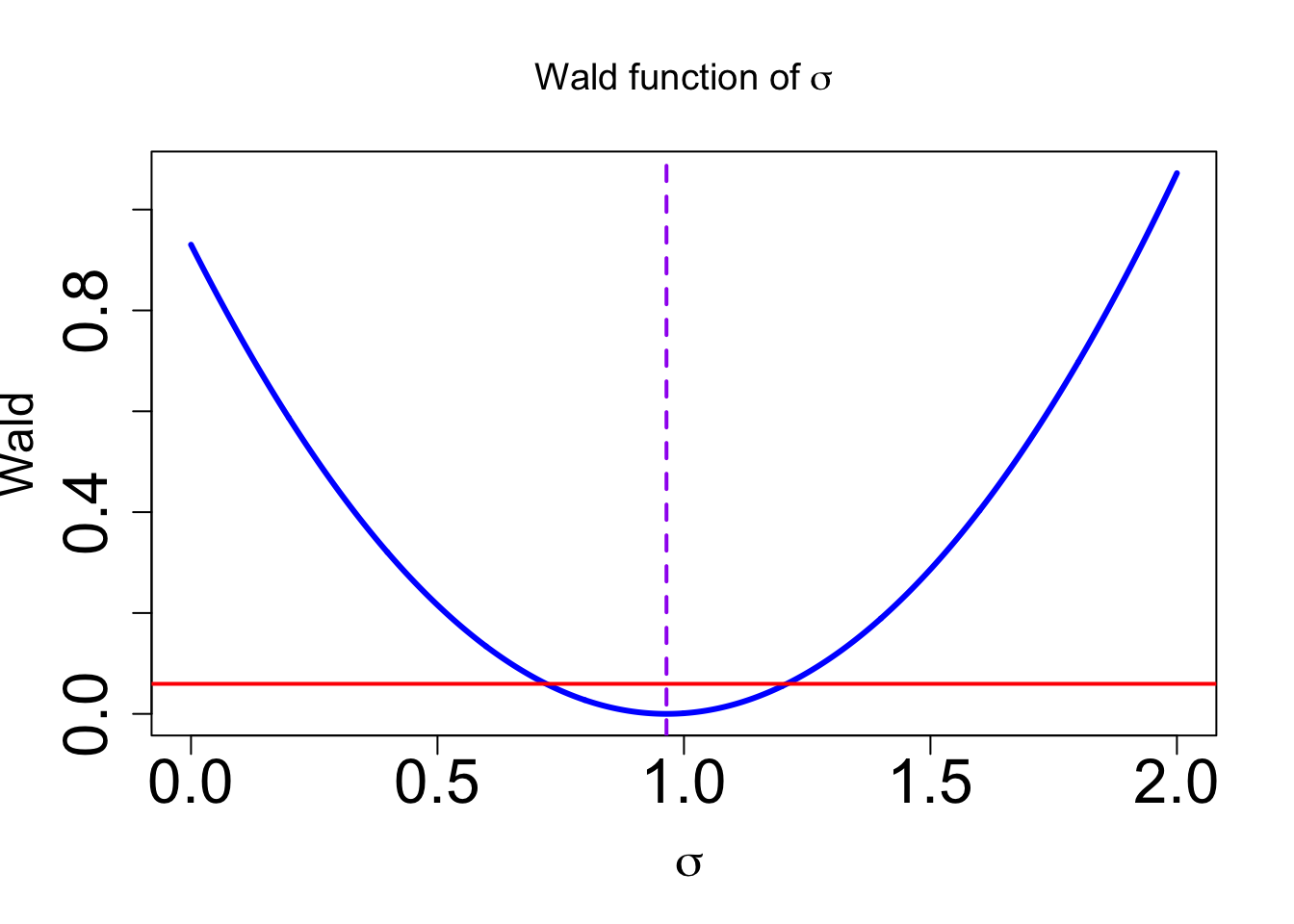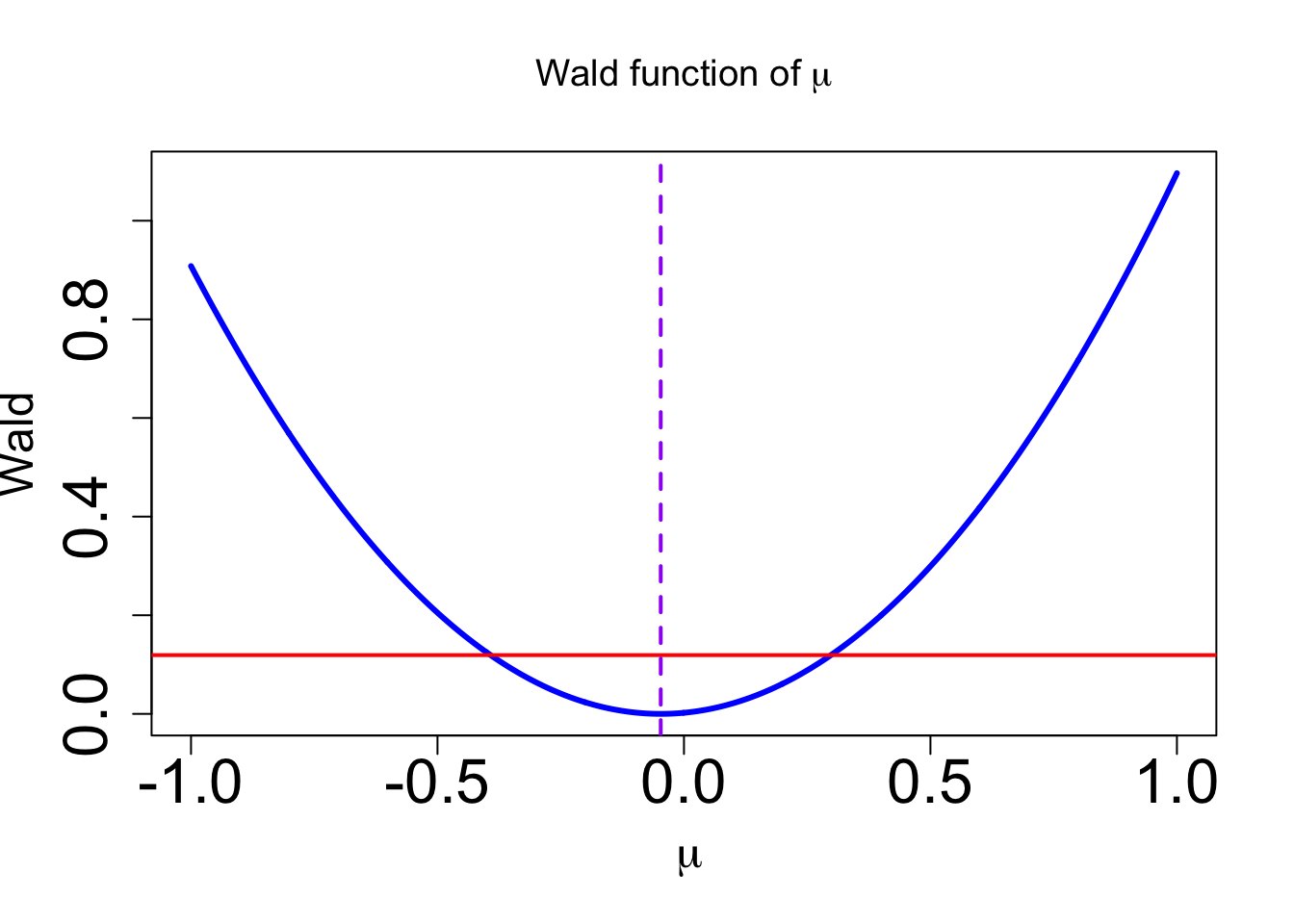# Second order approximation: Wald confidence intervals for the Normal distribution

Consider a second order approximation of $$\Lambda$$ around the MLE $$\widehat{\delta}$$: $\Lambda(\delta) = -2 \log R(\widehat{\delta} \mid {\bf x}) \approx \Lambda(\widehat{\delta})- 2\dfrac{R^{\prime}(\widehat{\delta} \mid {\bf x})}{R(\widehat{\delta} \mid {\bf x})} \left(\delta - \widehat{\delta}\right) + \dfrac{1}{2}\Lambda''(\widehat{\delta}) \left(\delta - \widehat{\delta}\right)^{2}.$ Using that the profile likelihood is maximised at $$\widehat{\delta}$$, then $$R(\widehat{\delta} \mid {\bf x})$$ and $$R'(\widehat{\delta} \mid {\bf x}) = 0$$ $\Lambda(\delta)\approx \dfrac{1}{2}\Lambda''(\widehat{\delta}) \left(\delta - \widehat{\delta}\right)^{2}.$ Compare this quantity with the quantity that we have studied in the context of asymptotic normality of the MLE, and notice that this is the square of such quantity. Using a formal argument, it is possible to prove that this quantity converges in distribution to a $$\chi^2_1$$. Thus, for large enough $$n$$, $\dfrac{1}{2}\Lambda''(\widehat{\delta}) (\delta - \widehat{\delta})^2 \stackrel{\cdot}{\sim}\chi^2_1.$ This provides an alternative way of approximating a confidence interval of level $$100(1-\alpha)\%$$, by calculating the values of $$\delta$$ that satisfy $$(\delta - \widehat{\delta})^2 \leq \dfrac{2Q_1(1-\alpha)}{\Lambda''(\widehat{\delta}) }$$.

The following R code shows how to use this method to calculate approximate $$95\%$$ confidence intervals for the parameters of the normal distribution. The second derivative is calculated using numerical methods for illustration purposes as this could be obtained explicitly from the expression of the profile likelihood obtained in the R Markdown Profile likelihood confidence intervals.

# $$95\%$$ Wald confidence interval for $$\sigma$$

# Delete memory
rm(list = ls())

# Required package
library(numDeriv)

# Simulated data from a normal with mean zero and variance 1
set.seed(123)
data = rnorm(n = 30, 0, 1 )
n = length(data)
s = sqrt(mean((data-mean(data))^2))
x.bar <- mean(data)
# MLE
MLE <- c(x.bar,s)
MLE
##  -0.04710376  0.96454162
# Profile likelihood of sigma
R.sigma = Vectorize( function(sigma) return( (s/sigma)^n*exp( 0.5*n*(1-(s/sigma)^2)) ) )

# Lambda function
Lambda <-  Vectorize( function(sigma) -2*log(R.sigma(sigma)) )
#----------------------------------------
# Wald approximation function for sigma
#-----------------------------------------

hess.sigma <-  hessian(Lambda , x = MLE)

# Threshold
lev <- 2*qchisq(0.95, df = 1)/hess.sigma

W.sigma = Vectorize( function(sigma) return( (sigma-MLE)^2 ))
# Visualising the profile likelihood of sigma
curve(W.sigma,0,2, n = 1000, lwd =3, col = "blue", cex.axis = 2, cex.lab = 1.5, main =  expression(paste("Wald function of ", sigma), ylim = c(0,1)),
xlab = ~sigma, ylab = "Wald")
abline(h = lev, lwd = 2, col = "red")
abline(v = MLE, lwd = 2, col = "purple", lty = 2)# 95% Confidence interval
W.sigmaC = Vectorize( function(sigma) return(W.sigma(sigma)-lev))

c(uniroot(W.sigmaC,c(0.6,0.8))$root,uniroot(W.sigmaC,c(1.1,1.5))$root)
##  0.7204769 1.2085908

## $$95\%$$ Wald confidence interval for $$\mu$$

# Delete memory
rm(list = ls())

# Required package
library(numDeriv)

# Simulated data from a normal with mean zero and variance 1
set.seed(123)
data = rnorm(n = 30, 0, 1 )
n = length(data)
s = sqrt(mean((data-mean(data))^2))
x.bar <- mean(data)
# MLE
MLE <- c(x.bar,s)
MLE
##  -0.04710376  0.96454162
# Profile likelihood of mu
R.mu = Vectorize( function(mu) return(  ( sum((data-x.bar)^2)/sum((data-mu)^2) )^(0.5*n)  ))

# Lambda function
Lambda <-  Vectorize( function(mu) -2*log(R.mu(mu)) )
#----------------------------------------
# Wald approximation function for mu
#-----------------------------------------

hess.mu <-  hessian(Lambda , x = MLE)

# Threshold
lev <- 2*qchisq(0.95, df = 1)/hess.mu

W.mu = Vectorize( function(mu) return( (mu-MLE)^2 ))
# Visualising the profile likelihood of sigma
curve(W.mu,-1,1, n = 1000, lwd =3, col = "blue", cex.axis = 2, cex.lab = 1.5, main =  expression(paste("Wald function of ", mu), ylim = c(0,1)),
xlab = ~mu, ylab = "Wald")
abline(h = lev, lwd = 2, col = "red")
abline(v = MLE, lwd = 2, col = "purple", lty = 2)# 95% Confidence interval
W.muC = Vectorize( function(mu) return(W.mu(mu)-lev))

c(uniroot(W.muC,c(-0.5,0.0))$root,uniroot(W.muC,c(0.0,0.5))$root)
##  -0.3922350  0.2980475